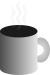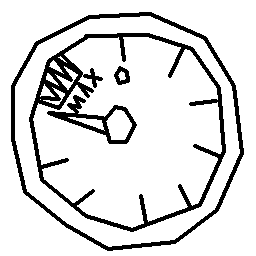#Coffee Space

Listen:

## C vs Java Large ArraysPreview Image

NOTE: Updated 01/12/2016.

### Introduction

I wanted to do a speed test to see how fast it is to process a large array for an idea I had for a neural network that is updated by some kernel method. I love and am very comfortable with Java, but am not completely against the idea of using another language if there is some benefit to doing so.

A language that is often pouted as being very fast is of course C - the reason why it is used in the Linux Kernel and appears in many other high end places. I decided to do a speed test in order to figure out whether the change would be worth it, so I devised a test to find out how much faster C would be that Java for processing large arrays.

There are some "gotchas" with this processing idea for the array - it has to be processed from the top to the bottom of the 2D array due to how the neurons speak to one another.

`````` ___ ___ ___
| L | C | R |
|___|___|___|
|   | N |   |
|___|___|___|
|   |   |   |
|___|___|___|``````

For the structure, `N` is equal to a summation of `A`, `B` and `C` - therefore they need to be processed first. This means that there is a limitation as to how parallelised the system can be made and care has to be taken in the threads, as will be seen in the below "Code" section.

### Code

For the first two examples, hopefully you will see that the code is very much similar in many respects and performance wise they have been given equal opportunity.

Also worth noting is that the 2 dimensional array has been unravelled into a 1 dimensional array for the purpose of speed. As the array is pretty large (~1 billion bytes) it makes a different.

#### C

``````#include <stdio.h>
#include <time.h>

#define size 30000
#define total (size * size)
#define cycles 100

char* grid;
clock_t start;
clock_t end;

int main(int argc, char** argv){
printf("\nBillion Neuron Speed Test\n");
printf("\nTotal neurons -> %i", total);
printf("\nPer side -> %i", size);
printf("\nCycles to test -> %i", cycles);
printf("\nInitialising variable grid...");
grid = (char*)malloc(sizeof(char) * total);
printf("\nGrid initialised!");
printf("\nExecuting speed test...");
start = clock();
int z;
int x;
for(z = 0; z < cycles; z++){
for(x = 0; x < total; x++){
grid[x] = x % 64;
}
}
end = clock();
printf("\nEnd of speed test!");
printf("\nTest took %d ms", ((end - start) / 1000));
printf("\n%f cycles per sec",  ((double)(cycles * 1000000) / (double)(end - start)));
}``````

#### Java

``````public class Speed{
private static final int size = 30000;
private static final int total = size * size;
private static final int cycles = 100;
private static byte[] grid;
private static long start;
private static long end;

public static void main(String[] args){
System.out.println("Billion Neuron Speed Test\n");
System.out.println("Total neurons -> " + total);
System.out.println("Per side -> " + size);
System.out.println("Cycles to test -> " + cycles);
System.out.println("Initialising variable grid...");
grid = new byte[total];
System.out.println("Grid initialised!");
System.out.println("Executing speed test...");
start = System.currentTimeMillis();
int z;
int x;
for(z = 0; z < cycles; z++){
for(x = 0; x < total; x++){
grid[x] = (byte)(x % 64);
}
}
end = System.currentTimeMillis();
System.out.println("End of speed test!");
System.out.println("Test took " + (end - start) + "ms");
System.out.println(((double)(cycles * 1000) / (double)(end - start)) + " cycles per sec");
}
}``````

``````import java.util.concurrent.atomic.AtomicInteger;

private static final int numThreads = 3;
private static final int size = 30000;
private static final int total = size * size;
private static final int cycles = 100;
private static AtomicInteger next = new AtomicInteger(0);
private static int cDone = numThreads;
private static AtomicInteger done = new AtomicInteger(0);
private static byte[] grid;
private static long start;
private static long end;

public static void main(String[] args){
System.out.println("Billion Neuron Speed Test\n");
System.out.println("Total neurons -> " + total);
System.out.println("Per side -> " + size);
System.out.println("Cycles to test -> " + cycles);
System.out.println("Initialising variable grid...");
grid = new byte[total];
System.out.println("Grid initialised!");
System.out.println("Executing speed test...");
start = System.currentTimeMillis();
/* NOTE: Size must be a multiple of what is being processed! */
int div = size / numThreads;
for(int x = 0; x < numThreads; x++){
threads[x] = new Speed2(div * x, div * (x + 1), 0, size);
}
if(done.get() == cDone){
/* Store future exit cycles */
/* As we have all threads waiting, set the count to zero */
next.set(0);
t.interrupt();
}
}
}
end = System.currentTimeMillis();
System.out.println("End of speed test!");
System.out.println("Test took " + (end - start) + "ms");
System.out.println(((double)(cycles * 1000) / (double)(end - start)) + " cycles per sec");
}

private int startX;
private int endX;
private int startY;
private int endY;

public Speed2(int startX, int endX, int startY, int endY){
this.startX = startX;
this.endX = endX;
this.startY = startY;
this.endY = endY;
}

public void run(){
int c;
int x;
int y;
int z;
for(c = 0; c < cycles; c++){
for(y = startY; y < endY; y++){
z = y * size;
for(x = startX; x < endX; x++){
grid[z + x] = (byte)(x % 64);
}
/* Update next counter */
next.incrementAndGet();
/* Wait for other threads to finish */
/* Wait in small loop */
}
}
/* Let main loop we are done */
done.incrementAndGet();
/* Allow main thread to perform clean-up service */
try{
/* This thread should not be asleep that long! */
}catch(InterruptedException e){
/* Do nothing */
}
}
}
}``````

### Results

#### C

``````\$ gcc Speed.c
\$ ./a.out
Billion Neuron Speed Test

Total neurons -> 900000000
Per side -> 30000
Cycles to test -> 100
Initialising variable grid...
Grid initialised!
Executing speed test...
End of speed test!
Test took 281309 ms
0.355481 cycles per sec``````

#### Java

``````\$ javac Speed.java
\$ java Speed
Billion Neuron Speed Test

Total neurons -> 900000000
Per side -> 30000
Cycles to test -> 100
Initialising variable grid...
Grid initialised!
Executing speed test...
End of speed test!
Test took 135388ms
0.7386178981889089 cycles per sec``````

``````\$ javac Speed2.java
\$ java Speed2
Billion Neuron Speed Test

Total neurons -> 900000000
Per side -> 30000
Cycles to test -> 100
Initialising variable grid...
Grid initialised!
Executing speed test...
End of speed test!
Test took 228783ms
0.43709541355782555 cycles per sec``````

#### [Update] C (optimised compile `-O3`)

``````\$ gcc -O3 Speed.c
\$ ./a.out
Billion Neuron Speed Test

Total neurons -> 900000000
Per side -> 30000
Cycles to test -> 100
Initialising variable grid...
Grid initialised!
Executing speed test...
End of speed test!
Test took 73882 ms
1.353493 cycles per sec``````

### Conclusion

Well... I knew the JIT is pretty cool, but I was not expecting a single Threaded Java application to out perform a C application (NOTE: Before optimising the source code.) of near equal code at all. This is very unexpected. I hope this is not because Java is taking some shortcuts as it knows the byte arrays are never read from - this was the purpose of setting the array to be some function as opposed to a pre-determined value such as zero (which Java may have skipped over or done something cool).

I did expect the multi-threaded Java code to be worse that the single thread, as you always pay the cost of the worst thread, meaning if any of them are paused to execute different tasks the whole program takes the performance hit. For even larger tasks this code may work much more efficiently.